## Solutions of Electricity Lakhmir Singh Manjit Kaur LAQ, SAQ, VSAQ, MCQ and HOTS Pg No. 38 Class 10 Physics24A. Define resistivity. Write an expression for the resistivity of a substance. Give the meaning of each symbol which occurs in it.

→ By definitions the resistivity of a material of a conductor is the resistance offered by a unit length and unit cross-section of the conductor. It is intrinsic property of material.

The expression for the resistivity isWhere m = mass of the electron
N= number density of electron
E = charge on the electron
Ƭ = relaxation time.

24B. State the SI unit of resistivity.

→ Resistivity is commonly represented by the Greek letter ρ (rho). The SI unit of electrical resistivity is the ohm-metre (Ω⋅m).

24C. Distinguish between resistance and resistivity.

→ The following points state the differences between Resistance and Resistivity:
ResistivityResistance
The resistivity of a material is the resistance of a wire of that material of unit length and unit cross-sectional area.Resistance is the opposition to the flow of electric current in a substance.
It is an intrinsic property.It is an extrinsic property.
The resistivity of a conductor is always same and is independent of its length or size.The resistance of a conductor is dependent on its length or size.
The unit of resistivity is ohm-metre.The unit of Resistance is ohm.

24D. Name two factors on which the resistivity of a substance depends and two factors on which it does not depend.

→ Resistivity of a substance depends on the nature of the substance and its temperature. It does not depend on the length or thickness of the conductor.

24E. The resistance of a metal wire of length 1 m is 26 Ω at 20° C. If the diameter of the wire is 0.3 mm, what will be resistivity of metal at that temperature?

→ We know,Multiple Choice Questions (MCQs)-Pg-27

25. The resistance of a wire of length 300 m and cross-section area 1.0 mm2 made of material of resistivity 1.0 x 10-7 Ω m is :
A. 2 Ω
B. 3 Ω
C. 20 Ω
D. 30 Ω26. When the diameter of a wire is doubled, its resistance becomes:
A. double
B. four times
C. one-half
D. one-fourth

→ When the diameter of a wire is doubled, its resistance becomes one-fourth.

27. If the resistance of a certain copper wire is 1Ω, then the resistance of a similar nichrome wire will be about:
A. 25 Ω
B. 30 Ω
C. 60 Ω
D. 45 Ω

→ Alloy of nickel, chromium, manganese and iron having a resistivity of about 60 times more than that of copper.

28. If the diameter of a resistance wire is halved, then its resistance becomes:
A. four times
B. half
C. one-fourth
D. two times

→ If the diameter of a resistance wire is halved, then its resistance becomes four times.

29. The resistivity of a certain material is 0.6 Ωm. The material is most likely to be:
A. an insulator
B. a superconductor
C. a conductor
D. a semiconductor

→ The resistivity of semiconductor like silicon and germanium is in between those of conductor and insulator.

30. When the area of cross-section of a conductor is doubled, its resistance becomes:
A. double
B. half
C. four times
D. one-fourth

→ When the area of cross-section of a conductor is doubled, its resistance becomes.

31. The resistivity of copper metal depends on only one of the following factors. This factor is :
A. length
B. thickness
C. temperature
D. area of cross-section

→ The resistivity of copper metal depends on temperature.

32. If the area of cross-section of a resistance wire is halved, then its resistance becomes :
A. one-half
B. 2 times
C. one-fourth
D. 4 times

→ If the area of cross-section of a resistance wire is halved, then its resistance becomes twice.

Questions Based on High Order Thinking Skills (HOTS)-Pg-27

33. A piece of wire of resistance 20 Ω is drawn out so that its length is increased to twice its original length. Calculate the resistance of the wire in the new situation.

→ We known that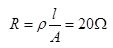Now if we stretch the same wire in such a way that its length get double. At the same time its area of cross-section decrease to half. So that34. The electrical resistivities of three materials P, Q and R are given below :
P 2.3 x 103 Ω m
Q 2.63 x 10-8 Ω m
R 1.0 x 1015 Ω m
Which material will you use making (a) electric wires (b) handle for soldering iron, and (c) solar cells? Give reasons for your choices.

→ (a) Material Q with resistivity 2.63×10-8 ohm-m can be used for making electric wires because it has very low resistivity.
(b) Material R with resistivity 1.0 ×1015 ohm-m can be used for making handle of soldering iron because it has very high resistivity.
(c) Material P with resistivity 2.3 × 103 ohm-m can be used for making solar cell because it is a semiconductor.

35. The electrical resisitivities of four materials A, B, C and D are given below :
A) 110 x 10-8 Ω m
B) 1.10 x 1010 Ω m
C) 01.0 x 10-8 Ω m
D) 2.3 x 103 Ω m
Which material is : (a) good conductor (b) resistor (c) insulator, and (d) semi-conductor ?

→ (a) Good conductor = C (10×10-8 ohm-m)
(b) Resistor= A (110×10-8 ohm-m)
(c) Insulator= B (1×1010 ohm-m)
(d) Semiconductor= D (2.3 ×103 ohm-m)

36. The electrical resistivities of five substances A, B, C, D and E are given below :
A) 5.20 x 10-8 Ω m
B) 2.60 x 10-8 Ω m
C) 10.0 x 10-8 Ω m
D) 1.70 x 10-8 Ω m
(a) Which substance is the best conductor of electricity? Why?
(b) Which one is better conductor: A or C? Why?
(c) Which substance would you advice to be used for making heating elements of electric irons? Why?
(d) Which two substances should be used for making electric wires? Why?

→ (a) E is the best conductor of electricity due to its least electrical resistivity.
(b) C, because its resistivity is lesser than that of A.
(c) B, because it has highest electrical resistivity
(d) C and E, because of their low electrical resistivities.

1. Give the law of combination of resistances in series.

→ When resistors are joined from end to end, it is called in series. In this case, the total resistance of the system is equal to the sum of the resistance of all the resistors in the system.

2. If five resistances, each of value 0.2 ohm, are connected in series, what will be the resultant resistance?

→ According to the law of combination of series connection
We know that3. State the law of combination of resistances in parallel.

→ When resistors are joined in parallel, the reciprocal of total resistance of the system is equal to the sum of reciprocal of the resistance of resistors.

4. If 3 resistances of 3 ohm each are connected in parallel, what will be their total resistance?

→ We know that in parallel combination, the reciprocal of total resistance is5. How should the two resistances of 2 ohms each be connected so as to produce an equivalent resistance of 1 ohm?

→ According to the question the resultant resistance is less than the individual resistances, so the resistances should be connected in parallel not in series.

6. Two resistances X and Y are connected turn by turn : (i) in parallel, and (ii) in series. In which case the resultant resistance will be less than either of the individual resistance?

→ The resultant resistance will be less than either of the individual resistance in parallel connection.

7. What possible values of resultant resistance one can get by combining two resistances, one of value 2 ohm and the other 6 ohm?

→ Here, R1=2 ohm, R2=6 ohm
When the resistors are arranged in parallel combination we get,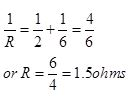Again, when the resistors are arranged in series combination we get
R = R1 + R2 = 2 + 6 = 8ohm

8. Show how you would connect two 4 ohm resistors to produce a combined resistance of (a) 2 ohms (b) 8 ohms.

→ When two 4 ohm resistors are connected in parallel then the equivalent resistance will be
1/Requivalent = 1/4 + 1/4 = 2/4 = 1/2
Or R = 2 ohms
When two 4 ohm resistors are connected in series then the equivalent resistance will be
Requivalent = 4 ohm + 4 ohm = 8 ohm

9. Which of the following resistor arrangement, A or B, has the lower combined resistance?→ Equivalent resistance in arrangement A is 10 ohm as this is in series.
And in arrangement B both 10 ohm and 1000 ohm are in parallel.
So, combined resistance of arrangement B is caculated as follows:
1/R = 1/10 + 1/1000 = (100+1)/1000
R = 1000/101 = 9.9 ohm
So, arrangement in B has lower combined resistance.

10. A wire that has resistance R is cut into two equal piece. The two parts are joined in parallel. What is the resistance of the combination?

→ As the wire is cut into two equal pieces. So resistance of each part is R/2.
The two parts are joined in parallel. So the equivalent resistance will be
1/Requivalent = 2/R + 2/R
1/Requivalent= 4/R
Requivalent = R/4

11. Calculate the combined resistance in each case:→ (a) Here both resistance i.e. R1 = 500 ohm, R2 = 1000 ohm are connected in series
So equivalent resistance R = R1 + R2 = 500 + 1000 = 1500ohm.
(b) Here both resistance i.e. R1=2ohm, R2=2ohm are connected in parallel
So equivalent resistance
1/R=1/R1+1/R2
1/R=1/2+1/2
R=1ohm
(c) Here resistance R1=4ohm and R2=4ohm are in parallel and R3=3ohm are in series
According to the given figure,
1/R=1/R1+1/R2
1/R=1/4+1/4
R=2ohm
Total resistance of the given circuit =R+R3
=2+3=5ohm.

12. Find the current in each resistor in the circuit shown below: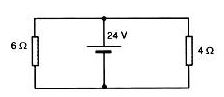→ Let R1=6Ὠ, R2=4Ὠ and V=24V
As the two resistances are connected in parallel. So the current across R1 will be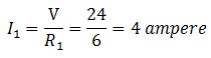And the Current across R2 will be13. Explain with diagrams what is meant by the “series combination” and “parallel combination” of resistances. In which case the resultant resistance is: (i) less, and (ii) more, than either of the individual resistances?

→ In a circuit, resistors are connected end-to-end are said to be in series, if the same current
exists in all of them through a single path. When resistances are connected in series, their equivalent resistance is equal to the sum of the individual resistances.

R = R1 + R2 + R3 + - - - - -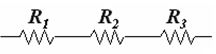When resistances are connected in parallel, the reciprocal of their equivalent resistance is equal to the sum of the reciprocals of the individual resistances.
1/R = 1/R1 + 1/R2 + 1/ R3 + - - - - -The resultant resistance is less than either of the individual resistances.

14. A battery of 9 V is connected in series with resistors of 0.2 Ω, 0.3 Ω, 0.4 Ω, 0.5 Ω and 12 Ω. How much current would flow through the 12 Ω resistor?

→ Here a battery of 9 V is connected in series with resistors of R1=0.2ohm, R2=0.4ohm, R3=0.3ohm, R4=0.5ohm, R5=12ohm,
So the resultant resistance = R1 + R2 + R3 + R4 + R5
R = 0.2+0.4+0.3+0.5+12=13.4ohm
As we know that
V= IR
Thus the current flow through 12ohm resistance will be equal to the current flowing across the whole circuit as in case of Series Connection same current flows through each resistance
∴ I = V/R
I = 9/13.4
I = 0.67amp.

15. An electric bulb of resistance 20Ω and a resistance wire of 4 Ω are connected in series with a 6 V battery. Draw the circuit diagram and calculate:
(a) total resistance of the circuit
(b) current through the circuit.
(c) potential difference across the electric bulb.
(d) potential difference across the resistance wire.(a) Here resistance are connected in series
Total resistance of the circuit = R1+R2=20+4=24Ω(ohm)
(b) According to ohm’s law.
V=IR
Therefore,
6V=I x 24Ω(ohm)
I=6 V /24 ohm = 0.25A(Ampere)
(c) Potential difference across the electric bulb
V1= IR1=0.25 X 20=5V
(d) Potential difference across the resistance wire
V2= IR2=0.25X4=1V

16. Three resistors are connected as shown in the diagram.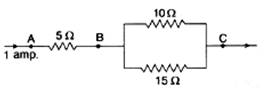Through the resistor 5 ohm, a current of 1 ampere is flowing
(i) What is the current through the other two resistors?
(ii) What is the p.d. across AB and across AC?
(iii) What is the total resistance?

→ (i) According to the figure.R1 and R2 are connected in parallel.
Let I be the current following in the circuit, which is equal to 1A. After passing through the resistance R3
Current I is divided into two part say I1 and I2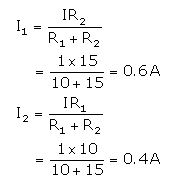(ii) Potential difference across AB = IR3 = 1 x 5 = 5V
As R1 and R2 are connected in parallel
So the equivalent resistance 1/Requivalent = 1/R1 +1/R2
1/Requivalent= 1/10 + 1/15
Requivalent= 6 ohm
Total resistance in the circuit
Rtotal= = 5+6 = 11 ohm
Potential difference across AC = IR = 1x11 = 11V

17. For the circuit shown in the diagram below:
What is the value of:(i) current through 6 Ω resistor?
(ii) potential difference across 12 Ω resistor?

→ The resistance of 6 ohm and 3 ohm are connected in series. Therefore, their net resistance can be calculated as
R= R1+R2
Here, R1= 6ohm
R2= 3ohm
so R= 6ohm +3ohm= 9 ohm
The current through 6 ohm resistor= current through line 1 = I= V/R =4/9=0.44V
(ii) The resistance of 12ohm and 3 ohm are connected in series. Therefore, their net resistance can be calculated as
R= R1+R2
Here, R1= 12ohm
R2= 3ohm
so R= 12ohm +3ohm= 15 ohm
The current through them = I= V/R =4/15
Potential difference across 12 ohm resistor= 4/15 ×12 =3.2

18. Two resistors, with resistances 5 Ω and 10 Ω respectively are to be connected to a battery of emf 6 V so as to obtain:
(i) minimum current flowing
(ii) maximum current flowing
(a) How will you connect the resistances in each case?
(b) Calculate the strength of the total current in the circuit in the two cases.

→ (i) For obtaining minimum current, the two resistors should be connected in parallel.
(ii) For obtaining maximum current, the two resistors should be connected in series.
(a) R1= 5 ohm, R2= 10 ohm and V = 6 V
For parallel combinationR= 10/3 ohm
Total current in the circuit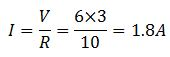For series combination
R=R1+R2
R=5+10=15 ohm
Total current in the circuit.(b) So the current in each case is 1.8 A and 0.4A in parallel and series circuit respectively.

19. The circuit diagram given below shows the combination of three resistors R1, R2 and R3 :Find:
(i) total resistance of the circuit.
(ii) total current flowing in the circuit.
(iii) the potential difference across R1.

→ (i) As shown in the figure, the resistor R2 and R3 are connected in parallel. Their total resistance is given by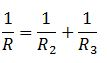Here R2= 3 ohm and R3=6 ohm
So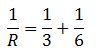R =2 ohm
The resistance R1 is in series with the equivalent resistance which are parallel.
Total resistance of the circuit = 2+4 ohms = 6 ohms
(ii) Total current flowing through the circuit=Potential difference/total resistance
Here p.d =12V, and total resistance = 6 ohms
I = 12/6 = 2amps
(iii) The potential difference across R1.
V = IR1=2×4 =8V

20. In the circuit diagram given below, the current flowing across 5 ohm resistor is 1 amp. Find the current flowing through the other two resistors.→ Given I= 1 A (Across 5 ohm)
R= 5 ohm
The potential drop across AB, V = I × R
or V= 5 ohm × 1A = 5V
In a parallel circuit, the potential difference across the ends of all resistors remains the same. Therefore the potential difference across 4 ohm and 10 ohm will be 5 V
The current flowing through the 4 ohm resistance
= V/R = 5/4 = 1.25 A
Current through 10 ohm resistor = V/R = 5/10 = 0.5 A

21. A resistor has a resistance of 176 ohms. How many of these resistors should be connected in parallel so that their combination draws a current of 5 amperes from a 220 volt supply line?

→ We Know according to Ohms Law that:-
V= IR, Where V= Voltage/Potential Difference          I= Current          R= Resistance According to Question :-V= 220V                                   I= 5A∴ Total Resistance of the Combination is :-Suppose x resistors should be connected in parallel to draw a current of 5A
for parallel combination:-
1/R = 1/R1 +1/R2+ 1/R3........1/RX(Where Resistance of each Resistor is 176Ω)
According to the question,
RCombination=176/x
⇒44=176/x( We know from above the total resistance of combination)
x=176/44=4 resistor
Hence 4 resistors each of 176Ω should be connected in parallel so as to draw the current of 5A from a 220 volt supply line.

22. An electric heater which is connected to 220 V supply line has two resistance coils A and B of 24 Ω resistance each. These coils can be used separately (one at a time), in series or in parallel. Calculate the current drawn when:
(a) only one coil A is used.
(b) coils A and B are used in series
(c) coils A and B are used in parallel.

→ (a) When only one coil, A is used
V= IR
220= 24I
I=9.2
(b) When coils A and B are used in series.
Total resistance R= RA +RB = 24+24 = 48ohms
I = V/R = 220/48
=4.58amps
(c) When coils A and B are used in parallel.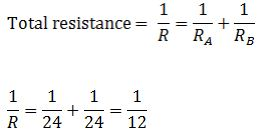R=12ohms
I = V/R = 220/12
=18.33amps

23. In the circuit diagram given below five resistances of 10 Ω, 40 Ω, 30 Ω, 20 Ω and 60 Ω are connected as shown to a 12 V battery.Calculate:
(a) total resistance in the circuit.
(b) total current flowing in the circuit.

→ (a) when three resistors are connected in parallel, the net resistance can be obtained as followed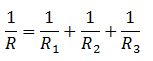The resistance of 30 ohm, 20 ohm and 60 ohm are connected in parallel. therefore, the net resistance R will be---------------------- (1)
The resistance of 10 ohm and 60 ohm are connected in parallel. Therefore, the net resistance R will beNow R1 and R2 are connected in series
So total resistance in the circuit is R = 10+8 =18 ohm
(b) Total current flowing in the circuit24. In the circuit diagram given below, three resistors, R1, R2 and R3 of 5 Ω, 10 Ω and 30 Ω respectively are connected as shown.Calculate :
(a) current through each resistor.
(b) total current in the circuit.
(c) total resistance in the circuit.

→ (a) Let I1, I2 and I3 be the current flowing through the resistors of 5 ohm, 100 ohm and 30 ohm, respectively.
According to ohm's law V=IR
Here V= 12 V and R = 5 ohm
I = V/R
Current through R1 = V/R1 = 12/5 = 2.4 A
Current through R2 = V/R2 = 12/10 = 1.2 A
Current through R3 = V/R2 = 12/30 = 0.4 A
(b) Total current in the circuit =Current through R1 +Current through R2 +Current through R3
= 2.4 + 1.2 + 0.4 = 4 A
(c) Total resistance in the circuit = R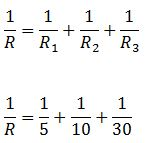R= 3 ohm

25. A p.d. of 4 V is applied to two resistors of 6 Ω and 2 Ω and 6 Ω connected in parallel. Calculate:
(a) the combined resistance
(b) the current flowing in the main circuit
(c) the current flowing in the 6Ω resistor.

→ (a) When two resistors are connected in series, their resultant resistance is given by
R= R1+R2
Here R1 = 6 ohm
R2= 2 ohm
Combined resistance, R = R1 + R2 = 6+2 = 8 ohm
(b) We know that the current, I= V/R
Here R = 8 ohm
V= 4V
I = 4/8 = 0.5 amp
(c) Potential difference across 6ohm resistor V= I x R1 = 0.5 x 6 = 3 V

26. A p.d. of 6 V is applied to two resistors of 3Ω and 6Ω connected in parallel. Calculate:
(a) the combined resistance
(b) the current flowing in the main circuit
(c) the current following in the 3Ω resistor

→ (a) ∵ the 3 Ω and 6 Ω resistance are in parallel, hence the eq. resistance =(b) I = V/Req = 4/2 = 2A
(c) Current Flowing in 3 Ω resistor =27. In the circuit shown below, the voltmeter reads 10 V.(a) What is the combined resistance?
(b) What current flows?
(c) What is the p.d. across 2 Ω resistor?
(d) What is the p.d. across 3 Ω resistor?

→ When two resistors are connected in series, their resultant resistance is given by
R= R1+R2
Here R1 = 2 ohm
R2= 3 ohm
Combined resistance, R = R1 + R2 = 2+3 = 5 ohm
(b) We know that the current, I= V/R
Here R = 5 ohm
V= 10V
I = 10/5 = 2 amp
(c) Potential difference across 2 ohm resistor V= I x R1= 2×2=4V
(d) Potential difference across 3 ohm resistor V= I x R2= 2×3=6V

28. In the circuit given below:(a) What is the combined resistance?
(b) What is the p.d. across the combined resistance?
(c) What is the p.d. across the 3 Ω resistor?
(d) What is the current in the 3Ω resistor?
(e) What is the current in the 6 Ω resistor?

→ (a)The resistors of 6 ohm and 3 ohm are connected in parallel. Therefore, their combined resistance can be calculated asR= 2 ohms
(b) The p.d. across the combined resistance, V= IR
Here I = 6A and combined resistance = 2 ohms.
So, V= 6 x 2 = 12 V
(c) In parallel connection potential difference remains constant, so Potential difference across 3 ohm resistor =12 V
(d) The current in the 3Ω resistor
I= V/R1
I= 12/3 =4A
(e) Current flowing through the 6 ohm resistor = V/R2 = 12/6 = 2 A

29. (a) 5 V battery is connected to two 20 Ω resistors which are joined together in series.
(a) Draw a circuit diagram to represent this. Add an arrow to indicate the direction of conventional current flow in the circuit.
(b) What is the effective resistance of the two resistors?
(c) Calculate the current that flows from the battery.
(d) What is the p.d. across each resistor?

→ (a)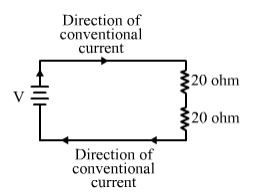(b) Since two resistors of 20 ohm are connected in series, the effective resistance will be
R= R1 +R2
Here, R1 = 20 ohm
R2 = 20 ohm
Therefore the effective resistance of the two resistors = 20+20 =40 ohm
(c) Current flowing through the circuit = I = V/R = 5/40 = 0.125 amps
(d) p.d. across each resistance = I x R = 0.125 x 20 = 2.5 V

30. The figure given below shows an electric circuit in which current flows from a 6V battery through two resistors.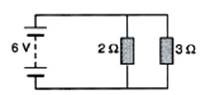(a) Are the resistors connected in series with each other or in parallel?
(b) For each resistor, state the p.d. across it.
(c) The current flowing from the battery is shared between the two resistors. Which resistor will have bigger share of the current
(d) Calculate the effective resistance of the two resistors.
(e) Calculate the current that flows from the battery.

→ (a) In the given figure resistors are connected in parallel.
(b) In a parallel arrangement, the voltage remains the same across each resistor i.e 6V
(c) Due to lower resistance 2 ohm have bigger share of current.
(d) The resistors of 2 ohm and 3 ohm are connected in parallel. Therefore, their combined resistance can be calculated asR= 1.2 ohm
(e) Current flowing through battery, I=V/R=6/1.2 = 5amps

31. A 4Ω coil and a 2 Ω coil are connected in parallel. What is their combined resistance? A total current of 3A passes through the coils. What current passes through the 2Ω coil?

→ The coils of the resistors 4 ohm and 2 ohm are connected in parallel. ThereforeR=4/3 ohm
Total current =3 A (given)
Potential difference V= 3×4/3=4V
Now current through 2Ω coil= V/2 =4/2 = 2A

32A. With the help of a circuit diagram, deduce the equivalent resistance of two resistances connected in series.Let the current in the circuit be I amperes and the battery be of strength V volts. Let the combined resistance of the three resistors be R ohms.
Therefore, according to Ohm's law, we have
V=IR ---- (i)
We know that when resistors are connected in series, the current with a battery of V volts.
V1=I×R1 ------- (ii)
V2 =I×R2 ------ (iii)
Let the potential difference across R1 is V1 and the potential difference across R2 is V2
V=V1+V2--------- (iv)
From equation (i),(ii), (iii) and (iv) we get.
IR= I×R1+ I×R2
R= R1+ R2

32B. Two resistances are connected in series as shown in the diagram:(i) What is the current through the 5 ohm resistance?
(ii) What is the current through R?
(iii) What is the value of R?
(iv) What is the value of V?

(i) Current through 5 ohm resistor
I= V/R
I = 10/5 = 2A
(ii) Two resistances are connected in series. So same current flow through the circuit,
So current flowing through R =2A
(iii) As we know that according to ohm's law
V=IR
So R= V/I = 6/2 = 3 ohm
(iv) Total V in the given circuit = V1+V2 = 10+6= 16 V

33A. With the help of a diagram, derive the formula for the resultant resistance of three resistors connected in series.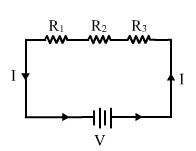Let the current be I amperes and the battery be of strength V volts. Let the combined resistance of the three resistors be R ohms.
Therefore, according to Ohm's law, we have
V= IR -----(i)
We know that when the resistors are connected in series, the current is the same in all the resistors. Therefore
V= V1 +V2+ V3 ------------------ (ii)
Let the current flowing through the whole circuit is I, and the equivalent resistance be R.
According to ohm's law
V= IR
V1= IR1 -------- (iii)
V2= IR2-------- (iv)
V3= IR3 ------ (v)
By using eq (i),(ii),(iii) ,(iv) and (v) we get
IR= IR1+ IR2+ IR3
R= R1+ R2+ R3

33B. For the circuit shown in the diagram given below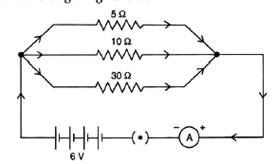Calculate:
(i) the value of current through each resistor.
(ii) the total current in the circuit.
(iii) the total effective resistance of the circuit.

→ (i) Here R1= 5 ohm, R2= 10 ohm and R3= 30 ohm
The value of current through each resistor is different as they are arranged in parallel connection.
So the current through 5 ohm resistor, I1 = V/R = 6/5 = 1.2A
So the current through 10 ohm resistor, I2 = V/R = 6/10 = 0.6A
So the current through 30 ohm resistor, I3 = V/R = 6/30 = 0.2A
(ii) The total current in the circuit = I= I1+ I2+ I3
1.2A + 0.6A + 0.2A = 2A
(iii) Total resistance in the circuit = R, As the circuit is in parallel connection, so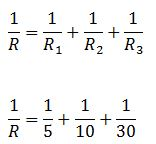R= 3 ohm

34A. With the help of a circuit diagram, obtain the relation for the equivalent resistance of two resistances connected in parallel.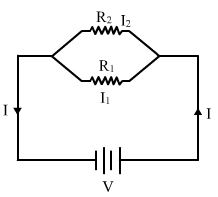Let the individual resistance of the two resistors be R1 and R2 and their combined resistance be R. Let the total current flowing in the circuit be I and strength of the battery be V volts. Then, from Ohm's law, we have: V= IR... (1)
We know that when resistors are connected in parallel, the potential drop across each resistance is the same. Therefore: =I=I1 + I2 = V/R1 + V/R2
I = V/(1 /R1 + 1/R2) ... (2)
From the equations (1) and (2) we have:
1/R = 1 /R1 + 1/R2

34B. In the circuit diagram shown below, find:
(i) Total resistance.
(ii) Current shown by the ammeter A→ (i) Let the total resistance of the circuit =R
As the connections are parallel. So the total resistance will be
1/R=1/R1+1/R2
R2=3+2=5ohms
R1=5 ohms
1/R=1/5+1/5
1/R=2/5
R=2.5ohms
(ii) Current shown by the ammeter A will be equal to the current following in the circuit.
I=V/R=4/(2.5)
=1.6amps

35A. Explain with the help of a labelled circuit diagram, how you will find the resistance of a combination of three resistors of resistances R1, R2 and R3 joined in parallel.Let the resistance of the three resistors be R1, R2 and R3, respectively. Let their combined resistance be R.
Let the total current flowing in the circuit be I and the strength of the battery be V. Then from Ohm's law, we have: V= IR------- (1)
We know that when the resistors are connected in parallel, the potential drop across each resistance is the same. Therefore:
I = I1+I2+I3
I = V/R1+ V/R2+ V/R3
1/R=1/R1+1/R2+ 1/R3
If two resistances are connected in parallel, then the resultant resistance will be
1/R=1/R1+1/R2+ 1/R3

35B. In the diagram shown below, the cell and the ammeter both have negligible resistance. The resistors are identicalWith the switch K open, the ammeter reads 0.6 A. What will the ammeter reading when the switch is closed?